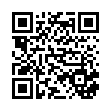# Basic Math 2007 F2 (1) .pdf

### File information

Original filename: Basic Math - 2007 - F2 (1).pdf
Author: Brian

This PDF 1.5 document has been generated by MicrosoftÂ® Word 2010, and has been sent on pdf-archive.com on 07/09/2017 at 07:48, from IP address 156.157.x.x. The current document download page has been viewed 158 times.
File size: 275 KB (12 pages).
Privacy: public file

Basic Math - 2007 - F2 (1).pdf (PDF, 275 KB)

### Document preview

Candidate’s No. ……………………………………
THE UNITED REPUBLIC OF TANZANIA
MINISTRY OF EDUCATION AND VOCATIONAL TRAINING
FORM TWO SECONDARY SCHOOL EXAMINATION, 2007
0041

BASIC MATHEMATICS

TIME: 2½ HOURS
INSTRUCTIONS
1.
2.
3.
4.
5.

This paper consists of sections A and B.
Answer ALL questions in both sections showing clearly all the working and
Write your examination number on the top right hand corner of every page.
Mathematical tables, geometrical instruments and graph papers may be used
where necessary.
Calculators and Cellphones are not allowed in the examination room.
FOR EXAMINER’S USE ONLY
QUESTION NUMBER
SCORE
INITIALS OF EXAMINER
1
2
3
4
5
6
7
8
9
10
11
12
13
14
15
16
17
18
19
20
21
22
23
24
25
TOTAL
This paper consists of 12 printed pages.

1

This and other free resources can be found at http://maktaba.tetea.org

Candidate’s No. ……………………………………
SECTION A (60 MARKS)
5

6

1.

Which is greater

2.

The average of scores in three subjects is 33. If the average of scores in two
subjects is 16. Find the score of the third subject.

3.

Estimate 521 to the nearest hundreds and 29 to the nearest tens. Hence find
the product of the estimations.

6

or 9?

2

This and other free resources can be found at http://maktaba.tetea.org

Candidate’s No. ……………………………………
4.

Find the length of ̅̅̅̅
𝐴𝐶 in the figure below if ̅̅̅̅
𝐵𝐷 = 5cm, ̅̅̅̅
𝐷𝐶 = 5cm and ̅̅̅̅
𝐷𝐸 =
3cm.

A

E

3cm
C

5.

5cm

D

5cm

B

Without using mathematical tables evaluate
1

2 log 5 + 4 log 2 − 3 log 64 .

3

This and other free resources can be found at http://maktaba.tetea.org

Candidate’s No. ……………………………………
6.

A rope of 18m and 80cm is to be divided into four equal parts. How long will

7.

Simplify (144 + 20) × 48 + 4 ÷ 2

8.

The area of a trapezium is 4000cm2. If one of the parallel sides is 80cm and
the height of the trapezium is 40cm, find the length of the other parallel side.

4

This and other free resources can be found at http://maktaba.tetea.org

Candidate’s No. ……………………………………
9.

Express 0.125 as a percentage.

10.

An equilateral triangle of sides a, b, and c has a perimeter of 105m. Find the
length of side c.

11.

If a:b = 4:9 and b:c = 3:7, evaluate a:c.

5

This and other free resources can be found at http://maktaba.tetea.org

Candidate’s No. ……………………………………
12.

The sum of two integers is 6 and their difference is 4. Find the integers.

13.

If

𝑎−2𝑏
𝑎+2𝑏

𝑎

= 2, calculate the value of 𝑏.

6

This and other free resources can be found at http://maktaba.tetea.org

Candidate’s No. ……………………………………
9𝑡 2 − 16𝑟 2 .

14.

Factorize completely

15.

Find the images of B (3, 4) under a reflection in the y – axis and x – axis.

16.

Find m if (1 ∗ 3) ∗ 𝑚 = 18, given that 𝑎 ∗ 𝑏 = 𝑎2 + 𝑏 2

7

This and other free resources can be found at http://maktaba.tetea.org

Candidate’s No. ……………………………………
17.

The sides of a rectangle are (2 − √3)cm and (2 + √3)cm. Find the length of its
diagonal.

18.

Simplify by rationalizing the denominator of

8

√5
√5+√3

This and other free resources can be found at http://maktaba.tetea.org

Candidate’s No. ……………………………………
19.

3

Given that sin 𝐴 = 5 where A is an acute angle.
Find the value of – cos 𝐴 + 1

20.

Find the solution of the following inequality and locate it on a number line.
|4𝑥 − 9| ≤ 3.

9

This and other free resources can be found at http://maktaba.tetea.org

#### HTML Code

Copy the following HTML code to share your document on a Website or Blog

#### QR Code### Related keywords